# The Wheat and Chessboard Story. The Power of Exponents.The exponent of a number tells us how many times the number has been multiplied by itself.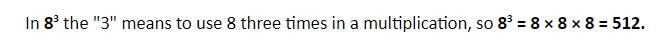Expressions that represent repeated multiplication of the same number are also called PowersThe topic of exponents is covered extensively in Grade 6, 8 and 9 IntoMath Lessons.

A very good illustration of exponents and their application can be found in the famous legend about the origin of chess.

When the inventor of chess showed his invention to the Emperor of India, he was very impressed by the invention.

He liked it so much, that he offered any reward the inventor could wish for.

The inventor said: “My wish is very simple.

There are 64 squares on the chessboard and for the

first square I wish only 1 grain of wheat,

for the second one I wish 2 grains,

for the third one I wish 4 grains,

for the fourth I wish 8 grains,

for the fifth I wish 16 grains

and so on for all the 64 squares”.

The emperor was not good at math and was surprised that the inventor seemed to have asked for such a small reward.

So the Emperor ordered his treasurer to give the inventor the wheat grains for all the squares as requested.

A few days later the Emperor asked his treasurer whether the chess inventor has been rewarded.

The treasurer informed the emperor that the reward turned out to be an astronomical sum, greater than all the wheat that could be gathered in many centuries.

This legend illustrates how powerful the exponents can be.

Let’s go through the legend once again, but this time, we will look at how the numbers were calculated to determine the amount of wheat grains.

The following chessboard contains 64 squares and illustrates how many grains of wheat each square the inventor asked for represents.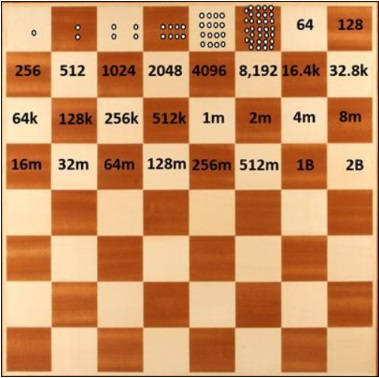If we look at the numbers on the squares, we can see that each subsequent square contains a number that is two times greater than the previous one.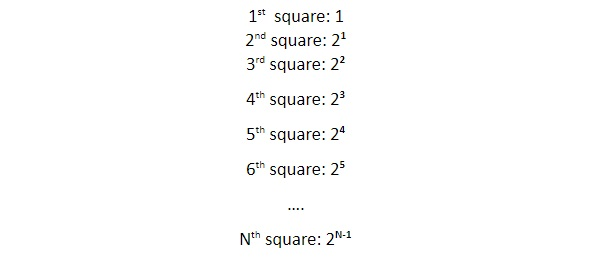So each subsequent number is 2 times greater than the previous one.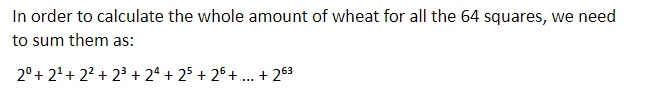Now if we look closer at the sequence, we can definitely conclude that the elements of the sequence represent geometric progression, which claims that any element in the geometric progression can be evaluated as: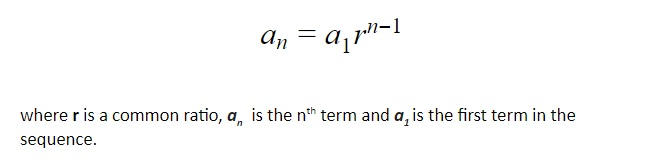The sum of all numbers in geometric progression is called Geometric Series.

We will look at the detailed explanation of geometric progression and series in the upcoming blog posts, but for now here is the formula for the geometric series:So, according to the above solution, which also involves operations in brackets, on the entire chessboard the number of wheat grains is equal to 18,446,744,073,709,551,615 weighing about 1,199,000,000,000 metric tons.

This is about 1645 times the global production of wheat in 2014!

See how the math can be fun and powerful?

0